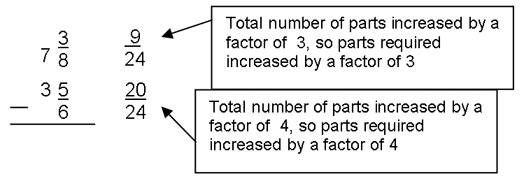# LAF Zone 5: Strategy Refining

The information below describes the key characteristics and teaching implications for Zone 5 of the Learning and Assessment Framework for Multiplicative Thinking (LAF), including:

## How to use this resource

There are eight zones in the LAF. If your students are across several zones, you should access information for each of the zones where they are located.

Where there is a reference to:

## Key characteristics

Systematically solves simple proportion and array problems, suggesting multiplicative thinking. For example:

• Butterfly House part e
• Packing Pots part a
• How Far part a .

May use additive thinking to solve simple proportion problems involving fractions. For example:

• School Fair part a
• Speedy Snail part b.

Able to solve simple, two-step problems using a recognised rule or relationship (for example, Fencing the Freeway Part A). However, finds this difficult for larger numbers. For example:

• Tables & Chairs parts k and l
• Tiles, Tiles, Tiles part c
• Stained Glass Windows part c.

Able to order numbers involving tens, ones, tenths and hundredths in supportive context. For example, Swimming Sports part A.

Able to determine all options in Cartesian product situations involving relatively small numbers, but tends to do this additively. For example:

• Canteen Capers part A
• Butterfly House parts 1 and I.

Beginning to work with decimal numbers and percent but unable to apply efficiently to solve problems. For example, Swimming Sports parts a and b, Computer Game part B.

Some evidence that multiplicative thinking being used to support partitioning. For example, Missing Numbers part B.

Beginning to approach a broader range of multiplicative situations more systematically.

## Teaching implications – consolidation and establishment

Consolidate or establish the ideas and strategies introduced or developed in the previous zone

## Teaching implications – introduction and development

Place-value

Introduce ideas and strategies for 5 digits and beyond if not already developed and decimal fractions to hundredths including renaming.

Multiplying and dividing by multiples of ten

Provide flexible, meaningful and efficient strategies for multiplying and dividing by multiples of ten. For example:

2.13 by 10, think: 21 ones and 3 tenths, 21.3.

The area idea and an expanded range of Cartesian product problems

Support multi-digit multiplication and formal recording (see Booker et al, 2004) through the area idea.

Introduce more efficient strategies for representing and solving an expanded range of Cartesian product problems involving three or more variables and tree diagram representations.

Formal terminology

Introduce terminology associated with multiplication and division such as:

• factor
• product
• divisor
• multiplier
• raised to the power of ….

Play the Factor Cross (pdf - 54.57kb) game.

Use calculators to explore what happens with repeated factors. For example:

• 4 × 4 × 4 × 4 …
• factors less than 1
• negative factors.

Renaming an expanded range of unrelated fractions

Introduce informal, partition-based strategies for renaming an expanded range of unrelated fractions as a precursor to developing an efficient, more formal strategy for generating equivalent fractions (see below).

For example:

explore using paper folding, diagrams and line models how sixths and eighths could be renamed as forty-eighths but they can also be renamed as twenty-fourths because both are factors of 24.

Generalisation for renaming fractions

If the number of equal parts (represented by the denominator) increases or decreases by a certain factor then the number of parts required (indicated by the numerator) increases or decreases by the same factor.

For example:

the total number of parts increased by a factor of 3, so parts required increased by a factor of 3.

Addition and subtraction of unlike fractions

Introduce written solution strategies for the addition and subtraction of unlike fractions.

For example:

think of a diagram showing sixths by eighths … forty-eighths… Is this the simplest? No, twenty-fourths will do, rename fractions by inspection9 twenty-fourths can’t take 20 twenty-fourths, trade 1 one for 24 twenty-fourths to get 6 and 33 twenty-fourths. Subtraction is then relatively straightforward

Link between multiplication and division and fractions

Explore the link between multiplication and division and fractions including decimals. For example:

3 pizzas shared among 4, 3 divided by 4 is 0.75 etc.

These links will help with understanding the fraction as operator idea. For example:

• &frac34; of 120
• 75% of \$48
• 250% of 458,239.

Use the Multiple Patterns (pdf - 18.5kb) task.

Establish benchmark equivalences. For example:

1 third = 331/3%.

Metacognitive strategies

Introduce strategies to support:

• problem comprehension
• strategy monitoring and checking
• interpretation of outcomes relevant to context.

For more information, see Siemon and Booker (1990) paper on Teaching and Learning For, About and Through Problem Solving (pdf - 462.19kb)

## Learning plans

The following learning plans have been developed for this zone, to support targeted teaching after students have been assessed and located on the LAF.

Zone 5 – Learning Plans Introducing (pdf - 77.05kb)